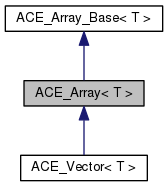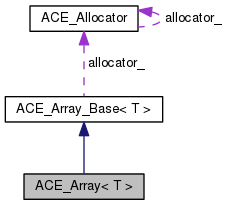ACE  6.2.3
ACE_Array< T > Class Template Reference

A dynamic array class. More...

`#include <Containers_T.h>`

Inheritance diagram for ACE_Array< T >:[legend]
Collaboration diagram for ACE_Array< T >:[legend]

## Public Types

typedef T TYPE

typedef ACE_Array_Iterator< T > ITERATORPublic Types inherited from ACE_Array_Base< T >
typedef T TYPE

typedef ACE_Array_Iterator< T > ITERATOR

typedef T value_type

typedef value_typeiterator

typedef value_type const * const_iterator

typedef value_typereference

typedef value_type const & const_reference

typedef value_typepointer

typedef value_type const * const_pointer

typedef ptrdiff_t difference_type

typedef ACE_Allocator::size_type size_type

## Public Member Functions

ACE_Array (size_t size=0, ACE_Allocator *alloc=0)
Dynamically create an uninitialized array. More...

ACE_Array (size_t size, const T &default_value, ACE_Allocator *alloc=0)
Dynamically initialize the entire array to the {default_value}. More...

ACE_Array (const ACE_Array< T > &s)
Copy constructor. More...

void operator= (const ACE_Array< T > &s)
Assignment operator. More...

bool operator== (const ACE_Array< T > &s) const
Equality comparison operator. More...

bool operator!= (const ACE_Array< T > &s) const
Inequality comparison operator. More...Public Member Functions inherited from ACE_Array_Base< T >
ACE_DECLARE_STL_REVERSE_ITERATORS ACE_Array_Base (size_type size=0, ACE_Allocator *the_allocator=0)
Dynamically create an uninitialized array. More...

ACE_Array_Base (size_type size, T const &default_value, ACE_Allocator *the_allocator=0)
Dynamically initialize the entire array to the default_value. More...

ACE_Array_Base (ACE_Array_Base< T > const &s)

void operator= (ACE_Array_Base< T > const &s)

~ACE_Array_Base (void)
Clean up the array (e.g., delete dynamically allocated memory). More...

T & operator[] (size_type slot)

T const & operator[] (size_type slot) const

int set (T const &new_item, size_type slot)

int get (T &item, size_type slot) const

size_type size (void) const
Returns the <cur_size_> of the array. More...

int size (size_type new_size)

size_type max_size (void) const
Returns the <max_size_> of the array. More...

int max_size (size_type new_size)

void swap (ACE_Array_Base< T > &array)

iterator begin (void)

iterator end (void)

const_iterator begin (void) const

const_iterator end (void) const

reverse_iterator rbegin (void)

reverse_iterator rend (void)

const_reverse_iterator rbegin (void) const

const_reverse_iterator rend (void) constProtected Member Functions inherited from ACE_Array_Base< T >
bool in_range (size_type slot) constProtected Attributes inherited from ACE_Array_Base< T >
size_type max_size_

size_type cur_size_

value_typearray_
Pointer to the array's storage buffer. More...

ACE_Allocatorallocator_
Allocation strategy of the ACE_Array_Base. More...

## Detailed Description

### template<class T> class ACE_Array< T >

A dynamic array class.

This class extends ACE_Array_Base, adding comparison operators.

Requirements and Performance Characteristics

• Internal Structure Dynamic array
• Duplicates allowed? Yes
• Random access allowed? Yes
• Search speed N/A
• Insert/replace speed O(1)
• Iterator still valid after change to container?
• In general, yes.
• If array size is changed during iteration, no.
• Frees memory for removed elements? No
• Items inserted by Value
• Requirements for contained type
1. Default constructor
2. Copy constructor
3. operator=
4. operator!=
ACE_Array_Base. This class inherits its operations and requirements.

## Member Typedef Documentation

template<class T>
 typedef ACE_Array_Iterator ACE_Array< T >::ITERATOR
template<class T>
 typedef T ACE_Array< T >::TYPE

## Constructor & Destructor Documentation

template<class T >
 ACE_Array< T >::ACE_Array ( size_t size = `0`, ACE_Allocator * alloc = `0` )
inline

Dynamically create an uninitialized array.

Initialize an empty array of the specified size using the provided allocation strategy.

template<class T>
 ACE_Array< T >::ACE_Array ( size_t size, const T & default_value, ACE_Allocator * alloc = `0` )
inline

Dynamically initialize the entire array to the {default_value}.

Initialize an array the given size placing the default_value in each index.

template<class T>
 ACE_Array< T >::ACE_Array ( const ACE_Array< T > & s )
inline

Copy constructor.

The copy constructor performs initialization by making an exact copy of the contents of parameter {s}, i.e., *this == s will return true.

## Member Function Documentation

template<class T>
 bool ACE_Array< T >::operator!= ( const ACE_Array< T > & s ) const
inline

Inequality comparison operator.

Compare this array with {s} for inequality such that {*this} != {s} is always the complement of the boolean return value of {*this} == {s}.

template<class T>
 void ACE_Array< T >::operator= ( const ACE_Array< T > & s )
inline

Assignment operator.

Assignment operator performs an assignment by making an exact copy of the contents of parameter {s}, i.e., *this == s will return true. Note that if the {max_size_} of {array_} is >= than {s.max_size_} we can copy it without reallocating. However, if {max_size_} is < {s.max_size_} we must delete the {array_}, reallocate a new {array_}, and then copy the contents of {s}.

template<class T>
 bool ACE_Array< T >::operator== ( const ACE_Array< T > & s ) const

Equality comparison operator.

Compare this array with {s} for equality. Two arrays are equal if their {size}'s are equal and all the elements from 0 .. {size} are equal.

The documentation for this class was generated from the following files: